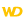HelpWLanguageWLanguage functionsControls, pages and windowsDrawing functionsPrefix syntaxDrawing functions on Image variablesRotationPresentationExampleEnd of drawingDrawing in PHPDrawing in Browser codeRelated examplesSee alsoAlphaBlendBackgroundBlurChangeModeCopyCropDominantImageColorDrawArcDrawBorderDrawChordDrawCircleDrawHaloDrawLineDrawPointDrawPolygonDrawPolylineDrawRectangleDrawRectangleGradientDrawRoundedRectangleDrawShadowDrawSliceDrawTextDrawTextRTFEncrustColorEndDrawingFillFontHorizontalSymmetryInvertColorModifyContrastModifyHSLModifyHueModifyLightnessModifyOpacityModifySaturationPenPixelColorPixelOpacityResizeRotationSaveBMPSaveGIFSaveJPEGSavePNGStartDrawingVerticalSymmetry
• End of drawing
• Drawing in PHP
• Drawing in Browser code
WINDEVWEBDEVWINDEV MobileOthers<Image variable>.Rotation (Function)
In french: <Variable Image>.Rotation
Rotates an image in a variable of type Image.Caution: A specific configuration is required to use this function in Linux. For more details, see The drawings.
Example
MyImage is Image = "Test.gif"
// Rotate Image variable by 45 degrees
Result1 is boolean
Result1 = MyImage.Rotation(45)
IF Result1 = False THEN Error("The operation failed")
IMG_MyDrawing = MyImage
Syntax

Performing a simple rotation

<Result> = <Image variable>.Rotation(<Rotation angle> [, <Options>])
<Result>: Boolean
• True if the rotation was performed,
• False otherwise.
<Image variable>: Image variable
Name of the Image variable to be used.
<Rotation angle>: Real
Rotation angle (in degrees).
• If the angle is positive, the image rotates clockwise.
• If the angle is negative, the image rotates counterclockwise.
<Options>: IntegerRotation options:
 drAdapt The image is flipped and enlarged. Then, its size is reduced to correspond to the initial image size. drDefault Enlarge the image (if necessary) so that it corresponds to the dimensions of rotated image.Constant used by default for the Image variables. drNoEnlarging The image is flipped but not enlarged: the image may be truncated.This parameter is not available.New in version 28This parameter is now available.
Remarks

End of drawing

If <Image variable>.EndDrawing and <Image variable>.Rotation are called in the same process, the image changes will not be displayed.
Related Examples:Unit examples (WINDEV): Image rotation [ + ] Image rotation from any point that will be used as rotation center.
Component: wd280pnt.dll
Minimum version required
• Version 24